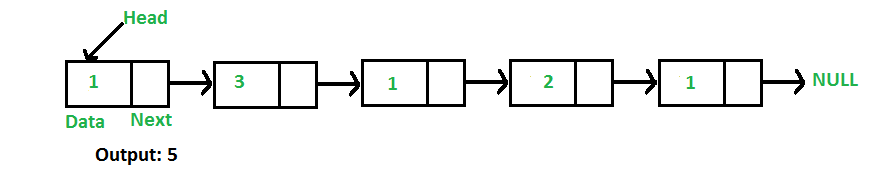# C++ Program For Finding Length Of A Linked List

• Last Updated : 27 Dec, 2021

Write a function to count the number of nodes in a given singly linked list.For example, the function should return 5 for linked list 1->3->1->2->1.

Iterative Solution:

```1) Initialize count as 0
2) Initialize a node pointer, current = head.
3) Do following while current is not NULL
a) current = current -> next
b) count++;
4) Return count ```

Following is the Iterative implementation of the above algorithm to find the count of nodes in a given singly linked list.

## C++

 `// Iterative C++ program to find length ``// or count of nodes in a linked list ``#include ``using` `namespace` `std;`` ` `// Link list node``class` `Node ``{ ``    ``public``:``    ``int` `data; ``    ``Node* next; ``}; `` ` `/* Given a reference (pointer to pointer)``   ``to the head of a list and an int, push ``   ``a new node on the front of the list. */``void` `push(Node** head_ref, ``int` `new_data) ``{ ``    ``// Allocate node``    ``Node* new_node =``new` `Node();`` ` `    ``// Put in the data``    ``new_node->data = new_data; `` ` `    ``// Link the old list off the ``    ``// new node``    ``new_node->next = (*head_ref); `` ` `    ``// Move the head to point to ``    ``// the new node``    ``(*head_ref) = new_node; ``} `` ` `// Counts no. of nodes in linked list``int` `getCount(Node* head) ``{ ``    ``// Initialize count ``    ``int` `count = 0; `` ` `    ``// Initialize current ``    ``Node* current = head;``    ``while` `(current != NULL) ``    ``{ ``        ``count++; ``        ``current = current->next; ``    ``} ``    ``return` `count; ``} `` ` `// Driver code``int` `main() ``{ ``    ``// Start with the empty list``    ``Node* head = NULL; `` ` `    ``// Use push() to construct list ``    ``// 1->2->1->3->1``    ``push(&head, 1); ``    ``push(&head, 3); ``    ``push(&head, 1); ``    ``push(&head, 2); ``    ``push(&head, 1); `` ` `    ``// Check the count function``    ``cout << ``"count of nodes is "` `<< ``             ``getCount(head); ``    ``return` `0; ``} `` ` `// This is code is contributed by rathbhupendra`

Output:

`count of nodes is 5`

Recursive Solution:

```int getCount(head)
1) If head is NULL, return 0.
2) Else return 1 + getCount(head->next) ```

Following is the Recursive implementation of the above algorithm to find the count of nodes in a given singly linked list.

## C++

 `// Recursive C++ program to find length``// or count of nodes in a linked list``#include ``using` `namespace` `std;`` ` `// Link list node``class` `Node ``{``    ``public``:``    ``int` `data;``    ``Node* next;``};`` ` `/* Given a reference (pointer to pointer) ``   ``to the head of a list and an int, ``   ``push a new node on the front of the list. */``void` `push(Node** head_ref, ``int` `new_data)``{``    ``// Allocate node ``    ``Node* new_node = ``new` `Node();`` ` `    ``// Put in the data``    ``new_node->data = new_data;`` ` `    ``// Link the old list off the ``    ``// new node ``    ``new_node->next = (*head_ref);`` ` `    ``// Move the head to point to ``    ``// the new node``    ``(*head_ref) = new_node;``}`` ` `// Recursively count number of ``// nodes in linked list ``int` `getCount(Node* head)``{``    ``// Base Case``    ``if` `(head == NULL) ``    ``{``        ``return` `0;``    ``}``    ``// Count this node plus the rest ``    ``// of the list``    ``else` `    ``{``        ``return` `1 + getCount(head->next);``    ``}``}`` ` `// Driver code``int` `main()``{``    ``// Start with the empty list``    ``Node* head = NULL;`` ` `    ``// Use push() to construct list``    ``// 1->2->1->3->1``    ``push(&head, 1);``    ``push(&head, 3);``    ``push(&head, 1);``    ``push(&head, 2);``    ``push(&head, 1);`` ` `    ``// Check the count function``    ``cout << ``"Count of nodes is "` `<< ``             ``getCount(head);``    ``return` `0;``}``// This is code is contributed by rajsanghavi9`

Output:

`Count of nodes is 5`

Please refer complete article on Find Length of a Linked List (Iterative and Recursive) for more details!

My Personal Notes arrow_drop_up